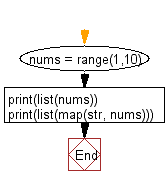﻿ Python: Generate and prints a list of numbers from 1 to 10 - w3resource# Python: Generate and prints a list of numbers from 1 to 10

## Python Basic - 1: Exercise-115 with Solution

Write a Python program to generate and prints a list of numbers from 1 to 10.

Expected output:
[1, 2, 3, 4, 5, 6, 7, 8, 9]
['1', '2', '3', '4', '5', '6', '7', '8', '9']

Sample Solution:

Python Code:

``````nums = range(1,10)
print(list(nums))
print(list(map(str, nums)))
``````

Sample Output:

```[1, 2, 3, 4, 5, 6, 7, 8, 9]
['1', '2', '3', '4', '5', '6', '7', '8', '9']
```

Flowchart:Python Code Editor:

Have another way to solve this solution? Contribute your code (and comments) through Disqus.

What is the difficulty level of this exercise?

Test your Programming skills with w3resource's quiz.

﻿

## Python: Tips of the Day

What is the difference between Python's list methods append and extend?

append: Appends object at the end.

```x = [1, 2, 3]
x.append([4, 5])
print (x)
```

Output:

```[1, 2, 3, [4, 5]]
```

extend: Extends list by appending elements from the iterable.

```x = [1, 2, 3]
x.extend([4, 5])
print (x)
```

Output:

```[1, 2, 3, 4, 5]
```

Ref: https://bit.ly/2AZ6ZFq